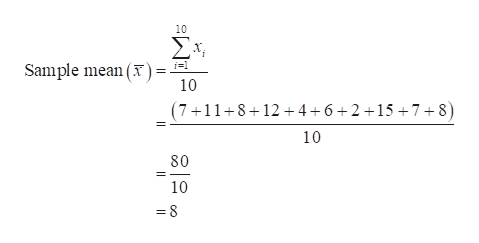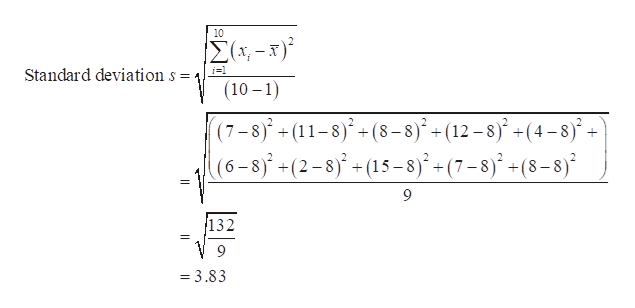Calculate the standard deviation of the data.7 11 8 12 4 6 2 15 7 8

Question

Calculate the standard deviation of the data.

7 11 8 12 4 6 2 15 7 8

Step 1

Standard deviation:

The standard deviation indicates by how much each observation differs from a central point represented by the mean. In general, the standard deviation increases with the increase in the distances between the individual observations and the mean of the data set.

The general formula for variance is,

Standard deviation s=Sqrt (sum of (xi-xbar)^2/n-1)

Step 2

Mean:

The mean of the sample data is:help_outlineImage Transcriptionclose10 Σ Sample mean ( 10 (7+11+8+12 4 6+2 15 7+8) 10 80 10 =8 fullscreen
Step 3

The standard deviation for ...help_outlineImage Transcriptionclose10 Σ-5 Standard deviation s = (10-1) (7-8)+(11-8)+(8-8)+(12-8(4-8 (6-8 - (2-8)- (15-sj°+(7=8j* + (8-8j* 9 132 = = 3.83 fullscreen

Want to see the full answer?

See Solution

Want to see this answer and more?

Our solutions are written by experts, many with advanced degrees, and available 24/7

See Solution
Tagged in

Hypothesis Testing